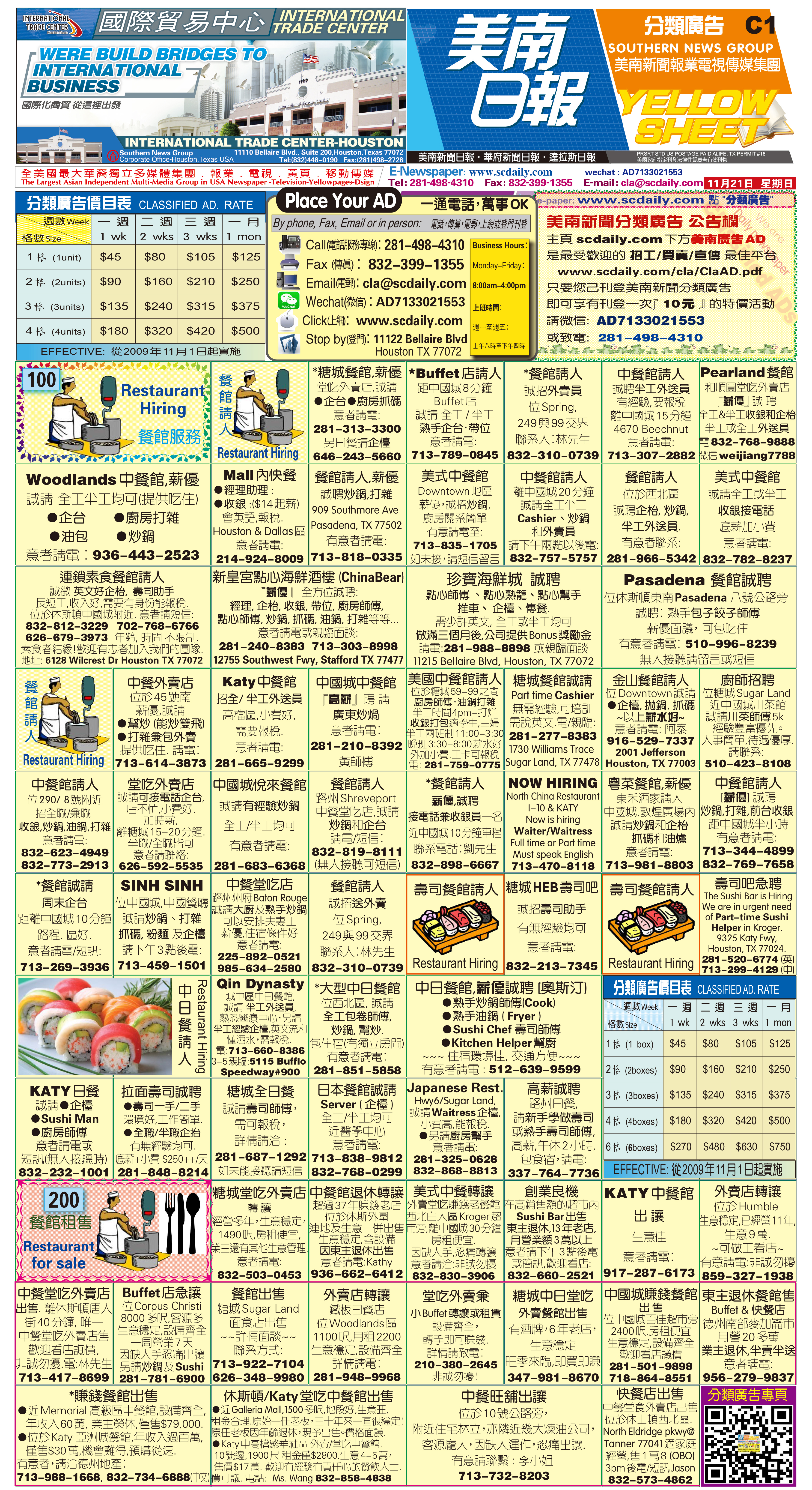211201 Epaper

 A Section B SectionC Section D Section211130 Epaper

 A Section B SectionC Section D Section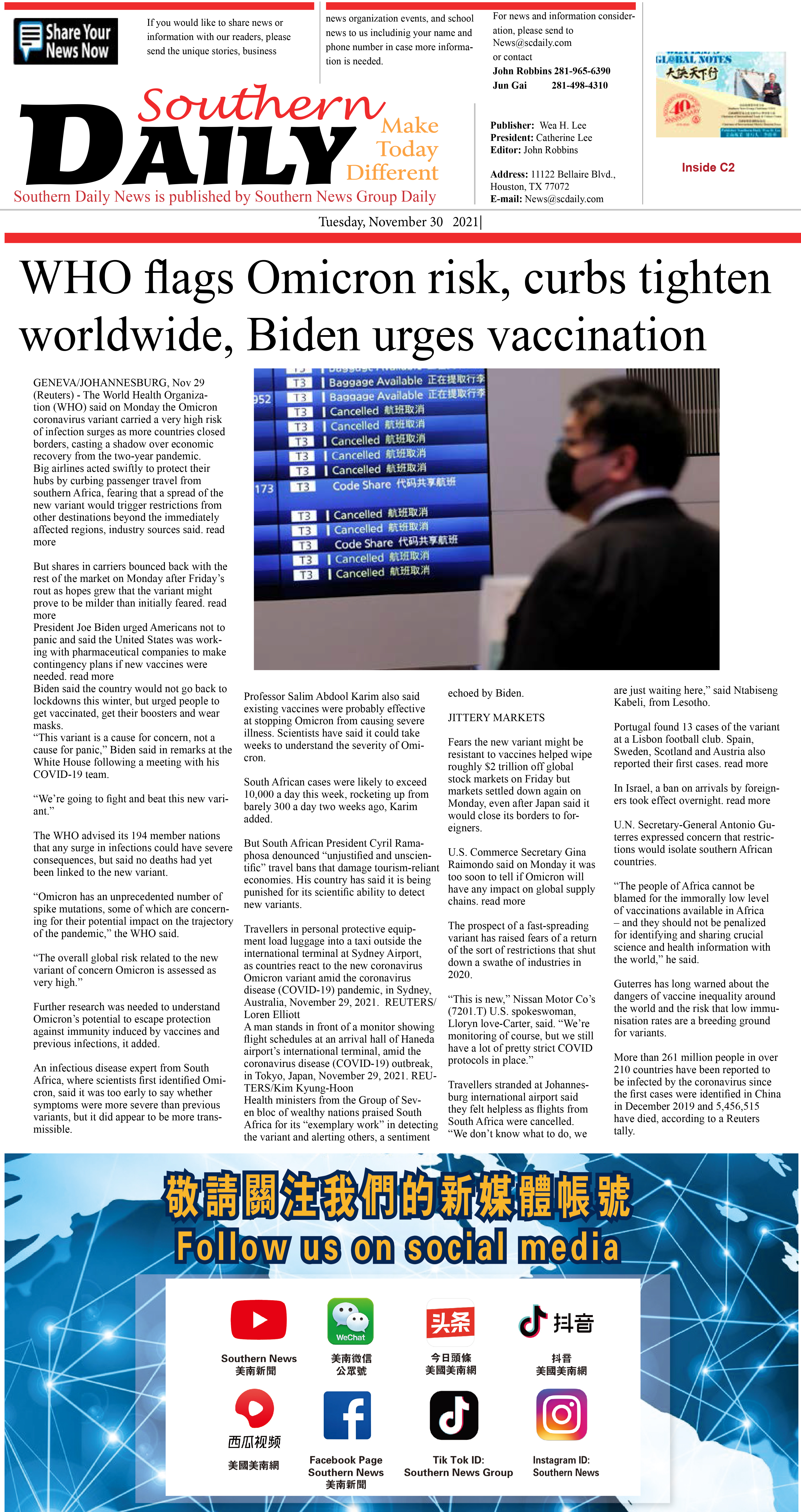211129 Epaper

 A Section B SectionC Section D Section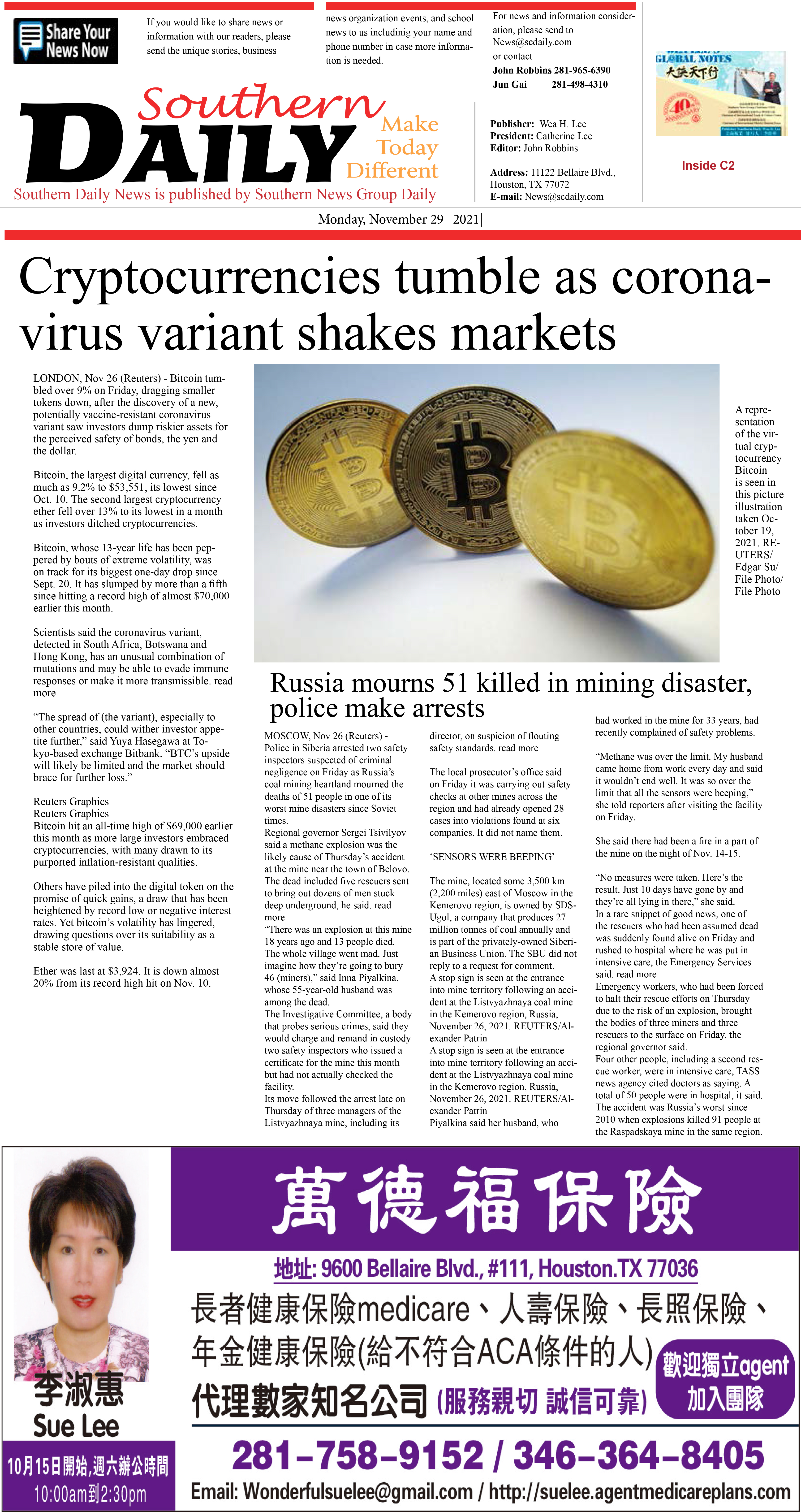211128 Epaper

 A Section B SectionC Section D Section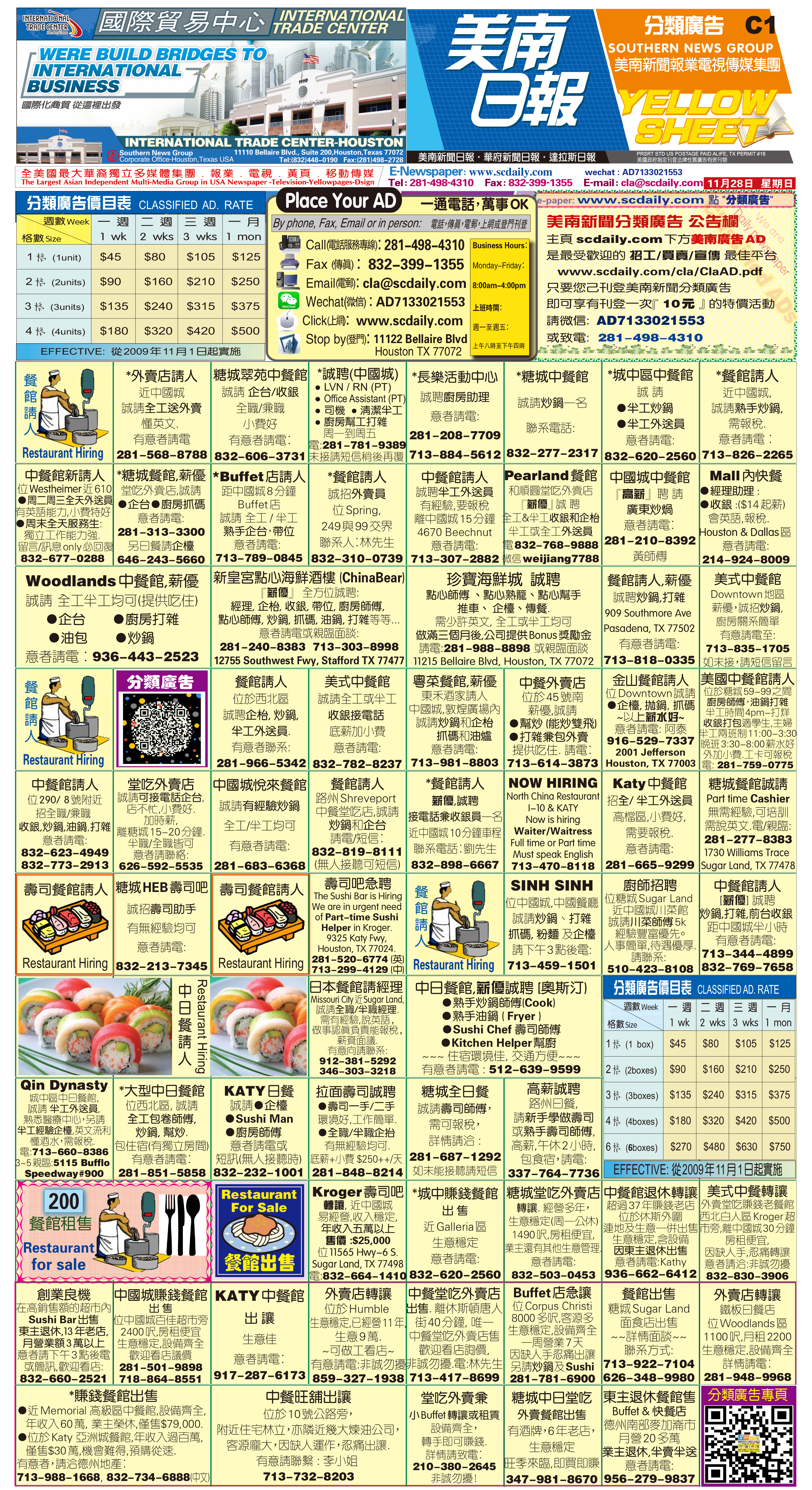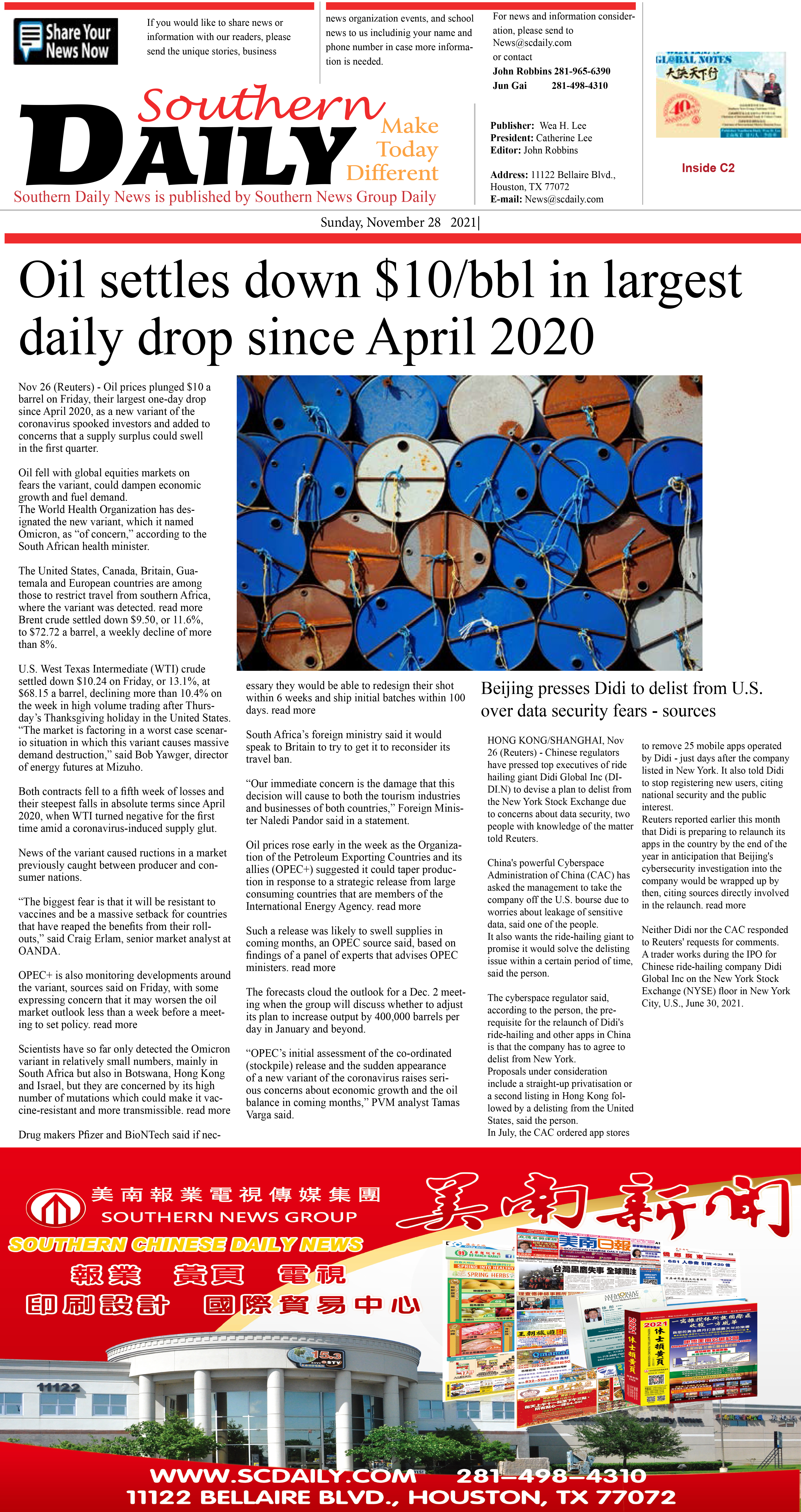211127 Epaper

 A Section B SectionC Section D Section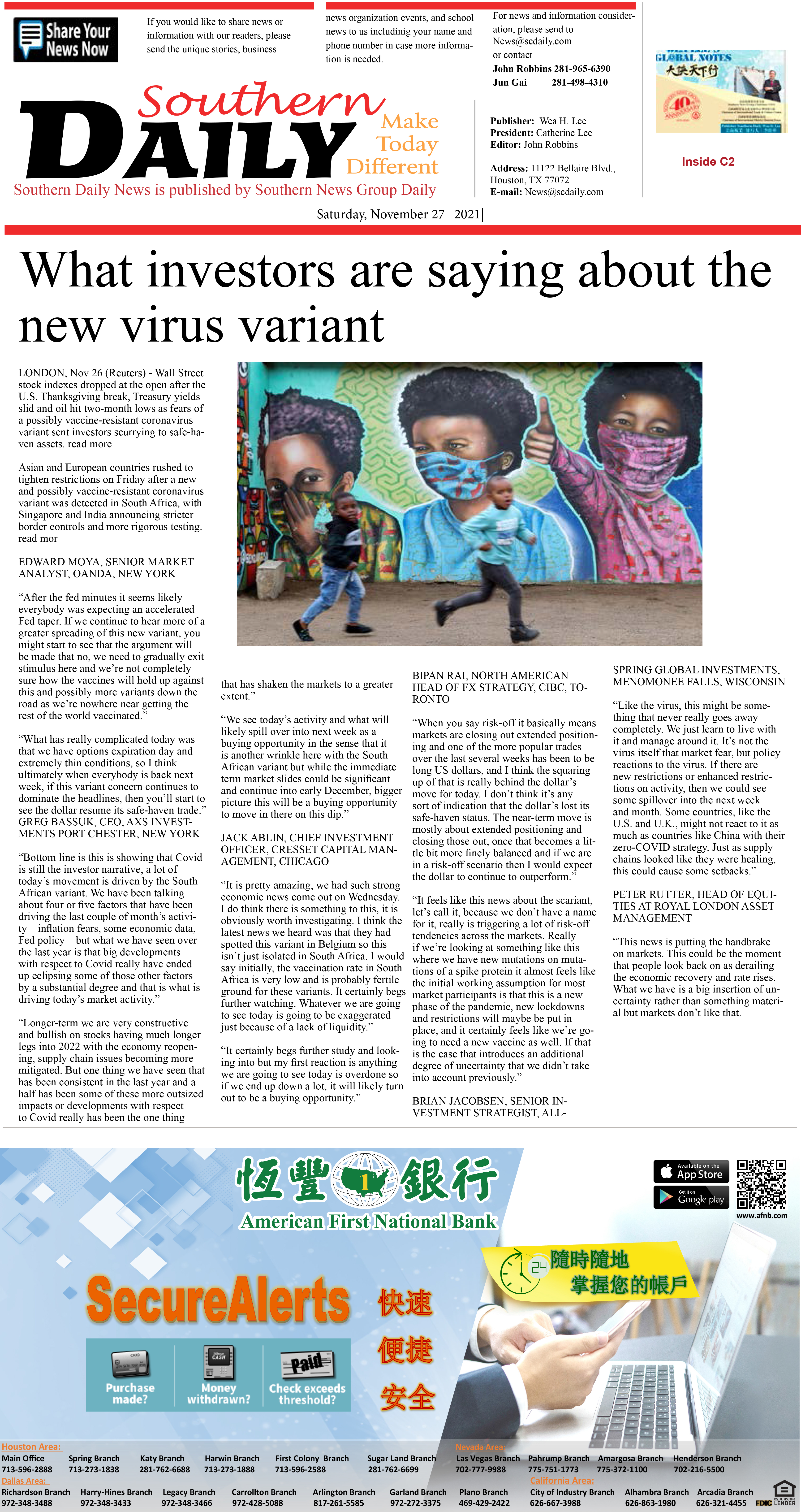211126 Epaper

 A Section B Section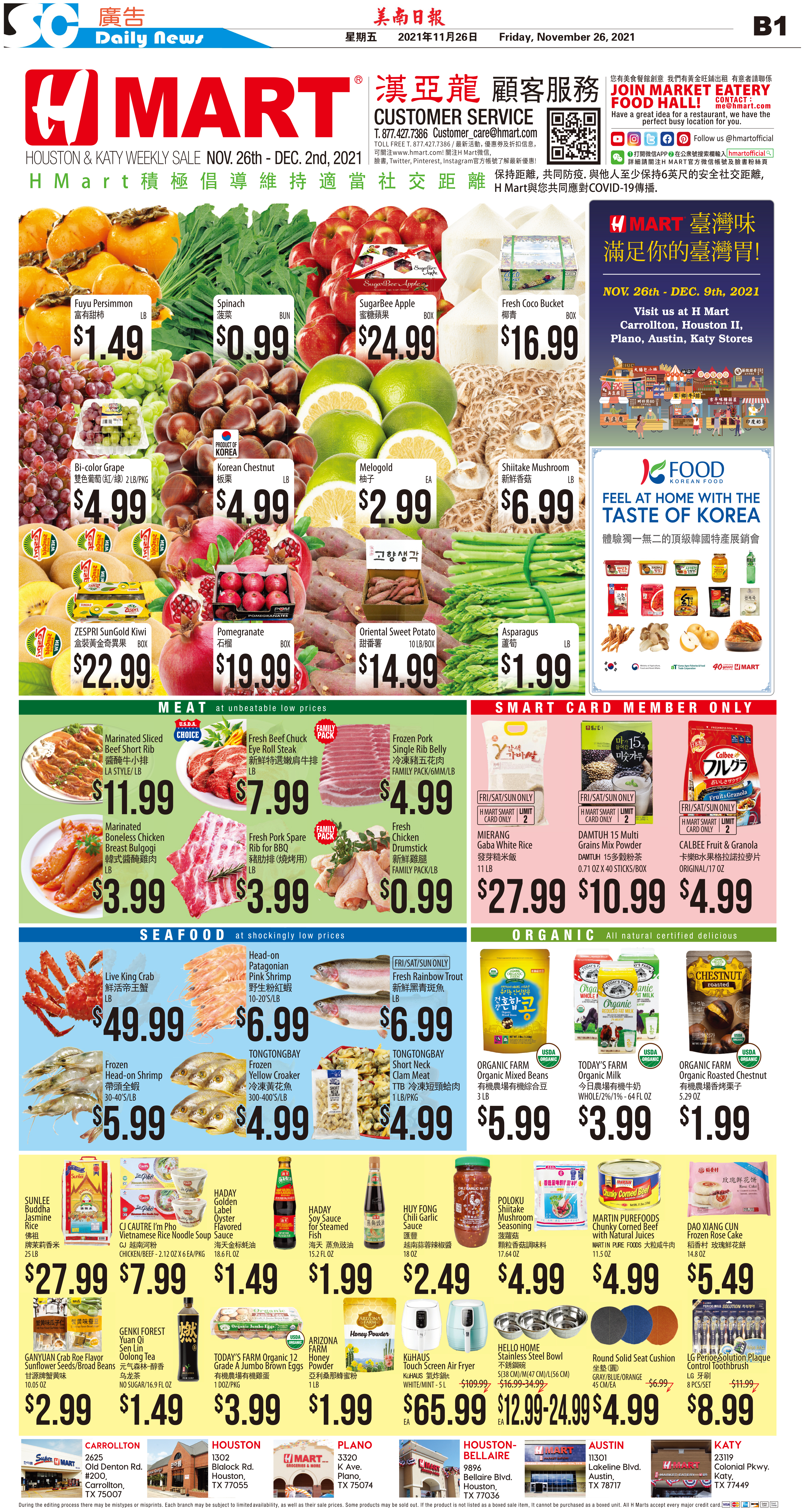C Section D Section211125 Epaper

 A Section B SectionC Section D Section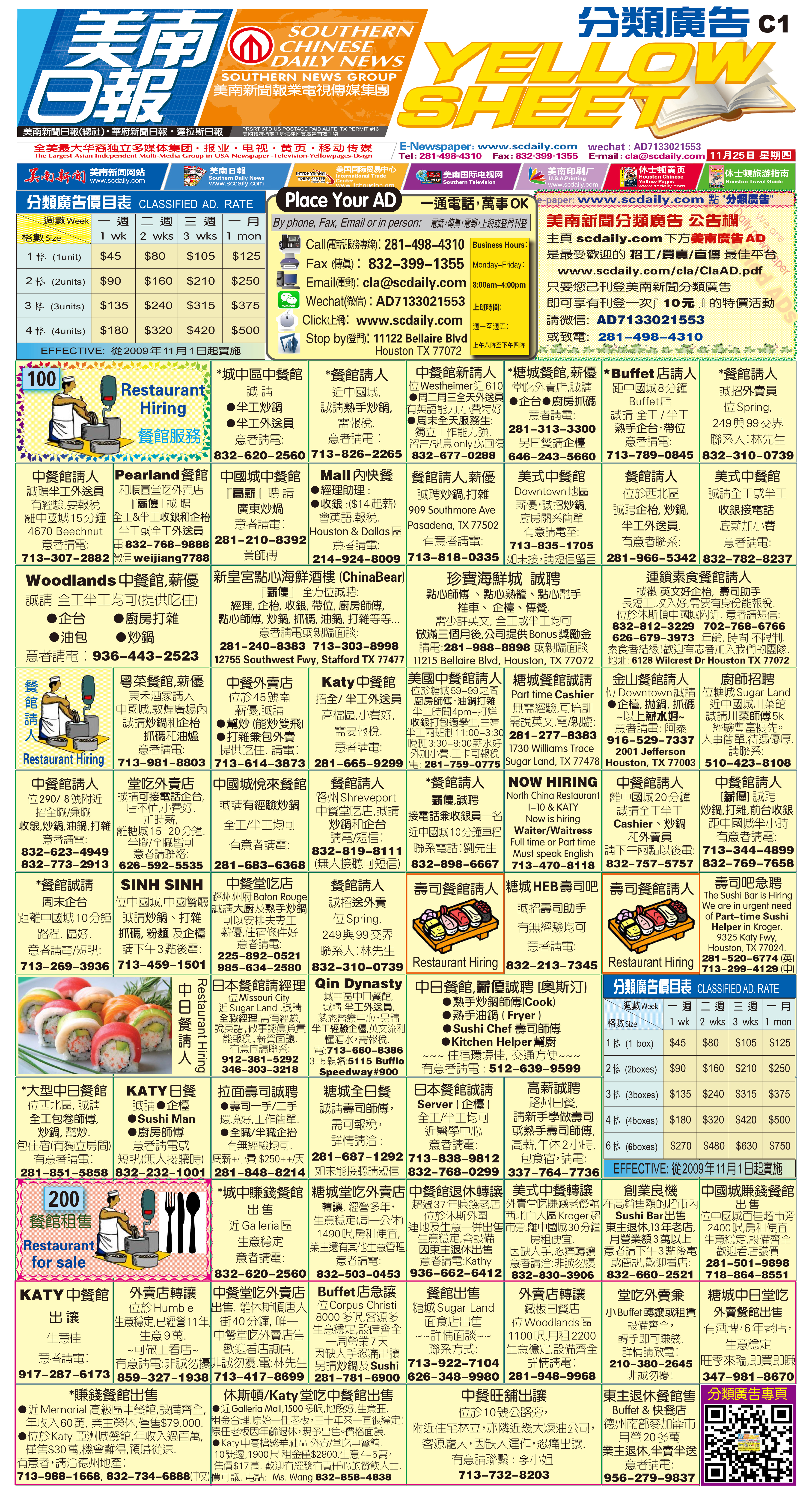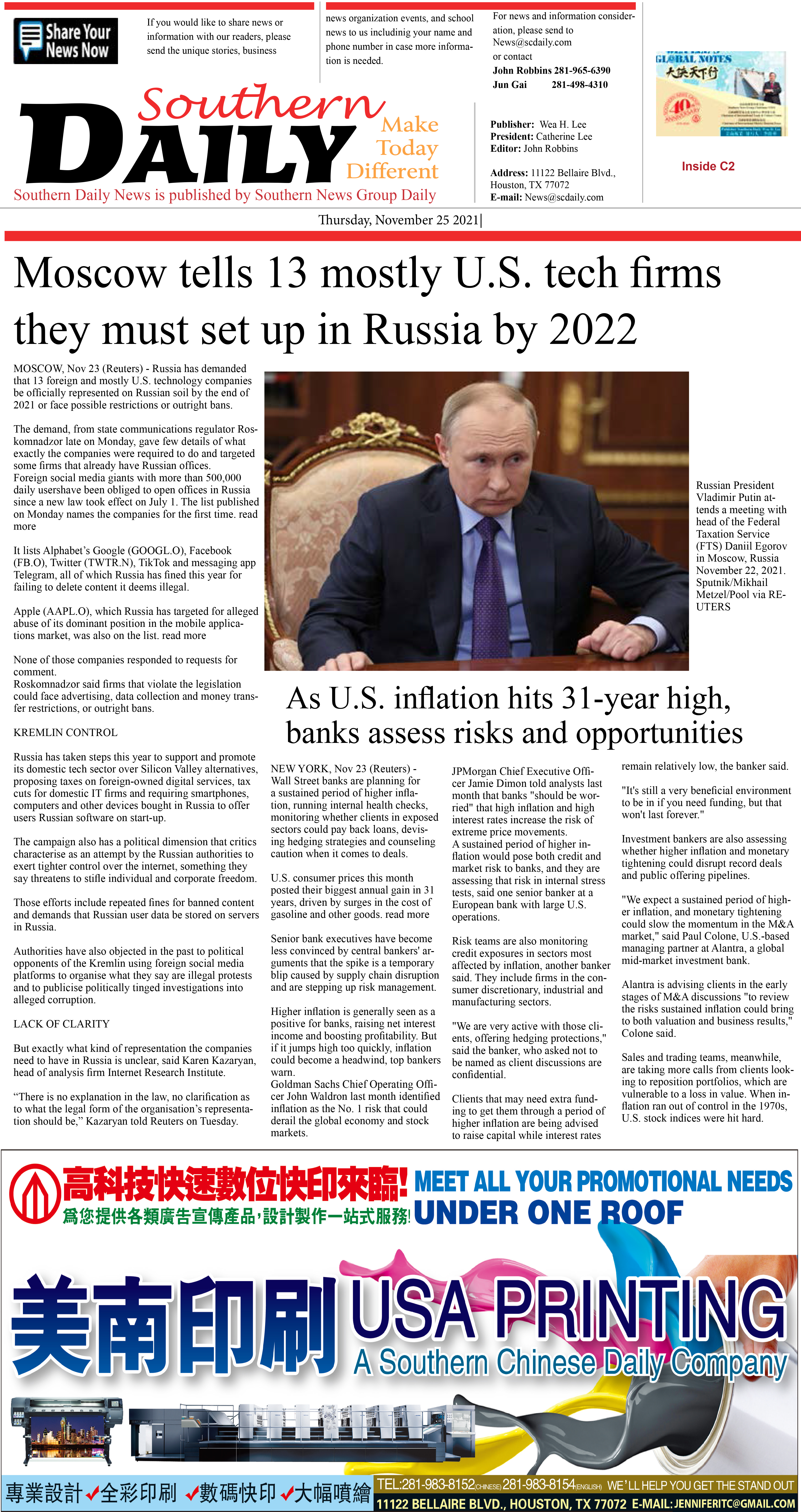211124 Epaper

 A Section B SectionC Section D Section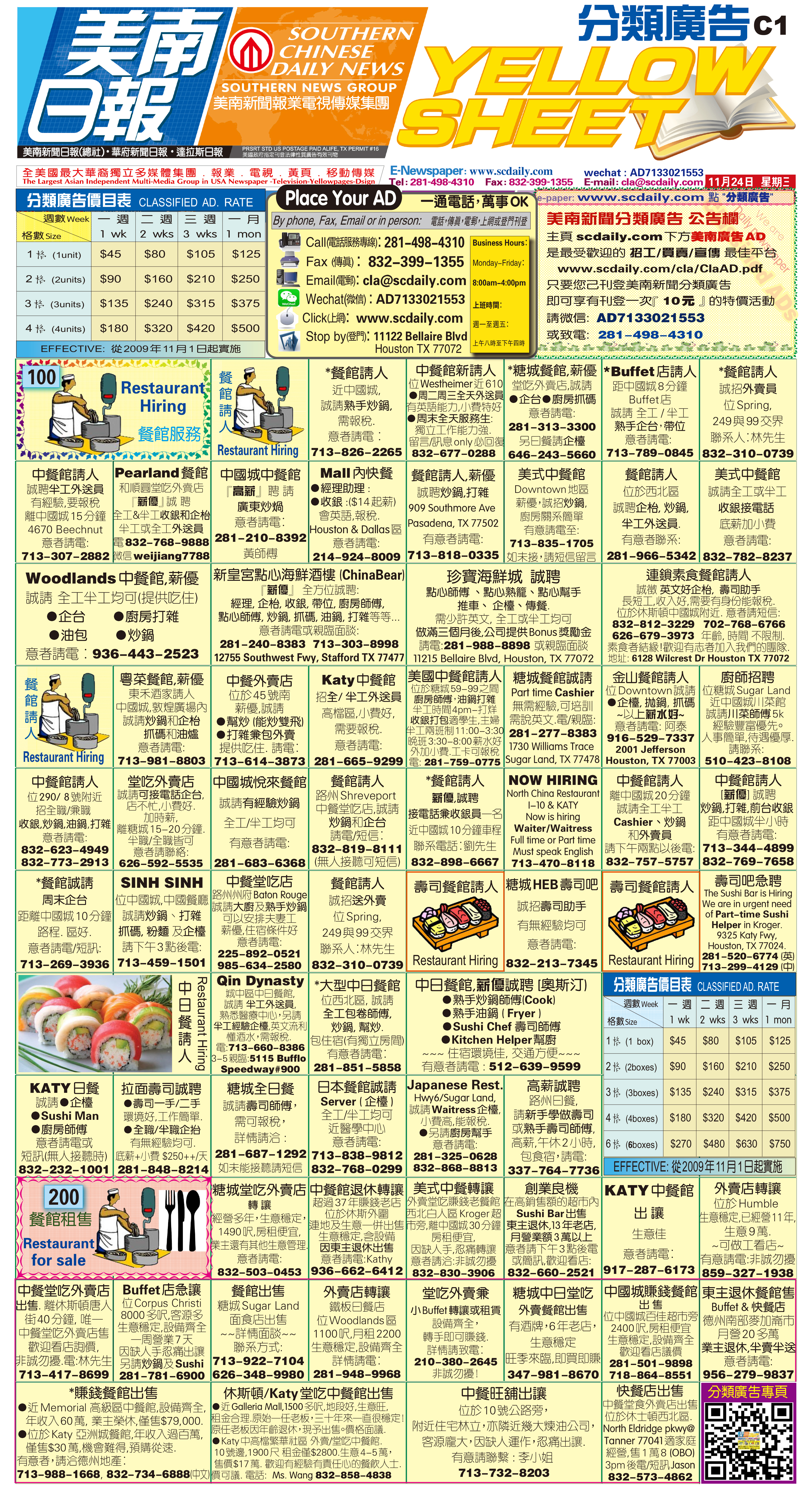211122 Epaper

 A Section B SectionC Section D Section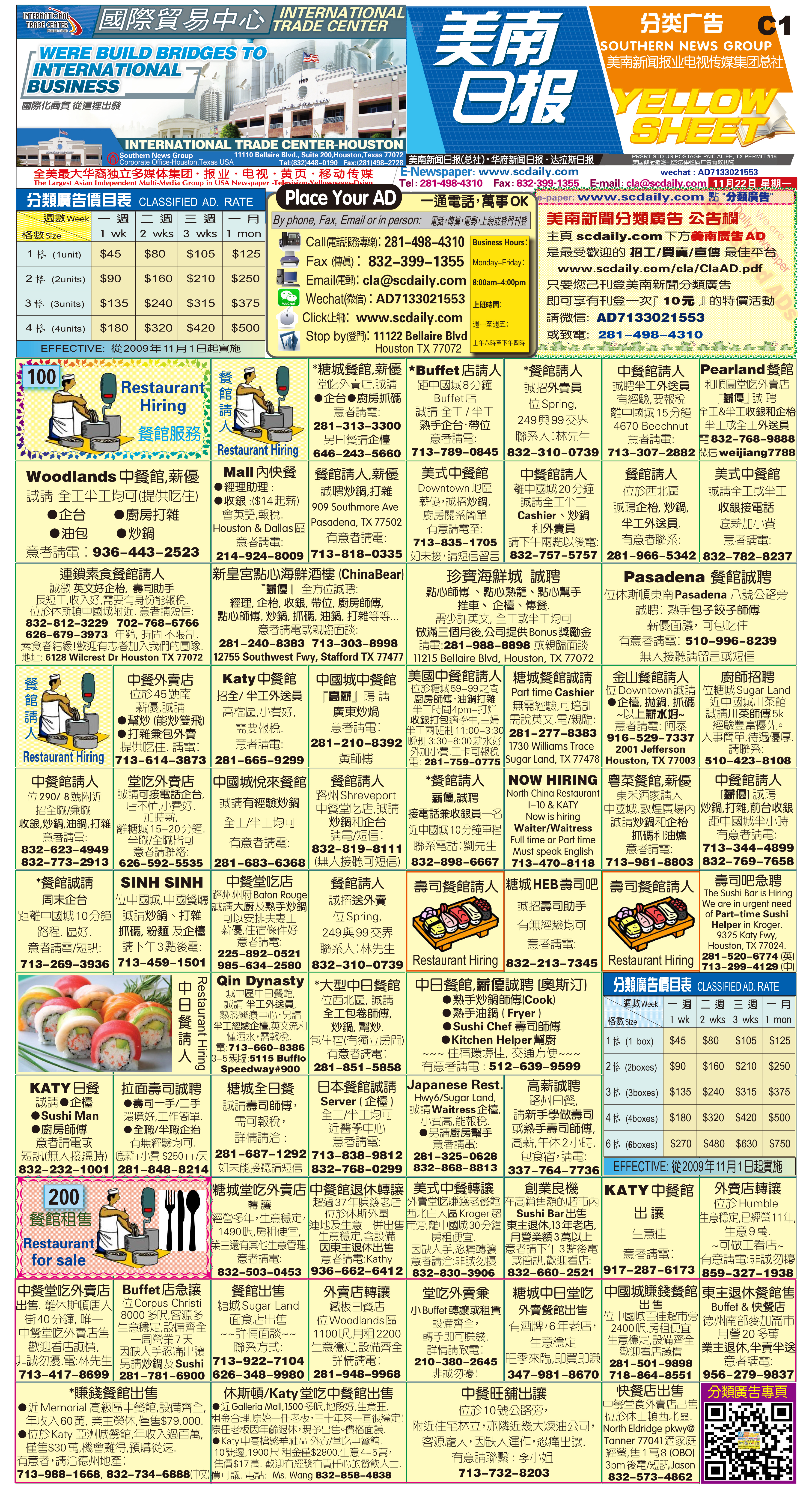211123 Epaper

 A Section B SectionC Section D Section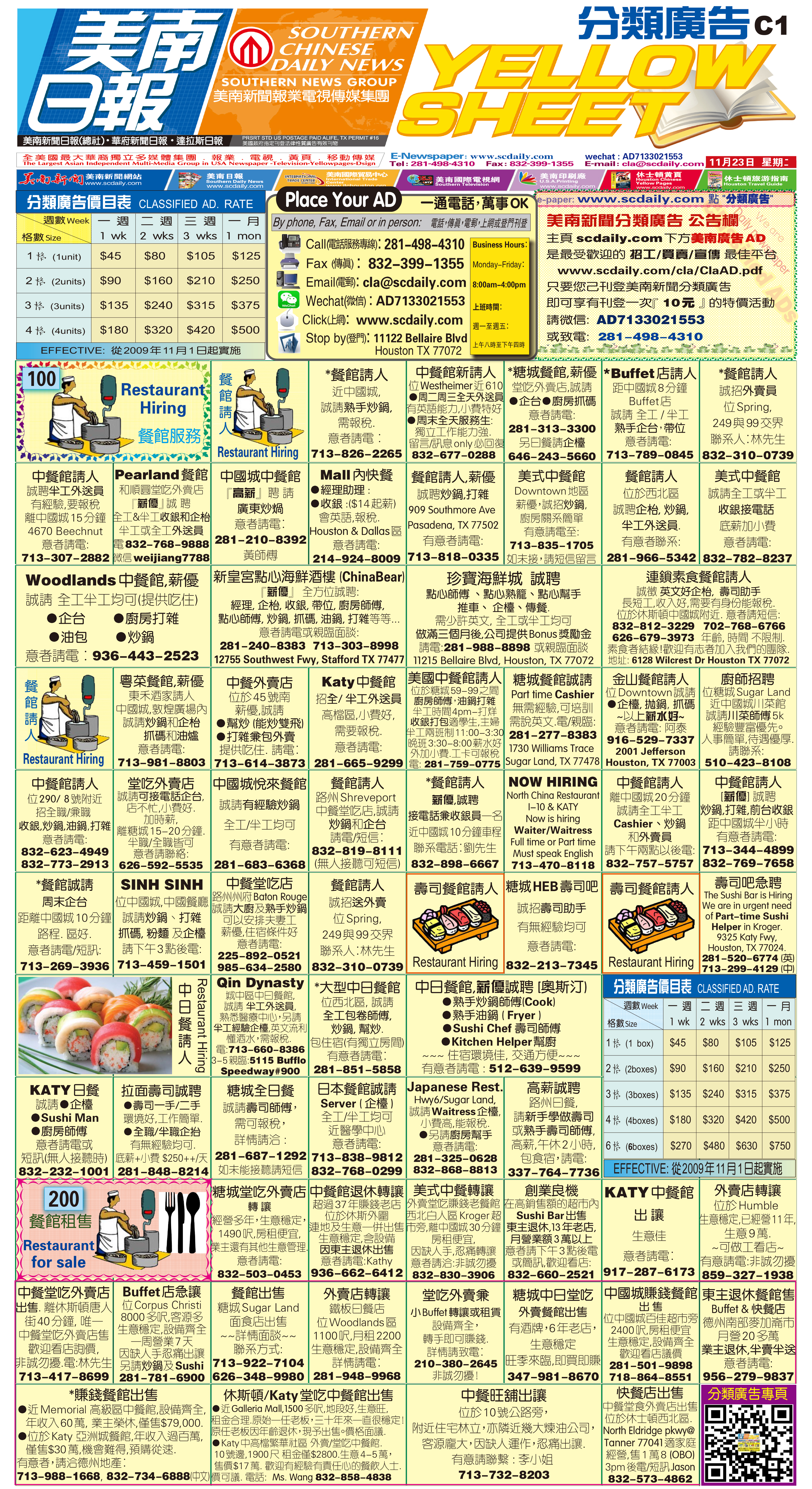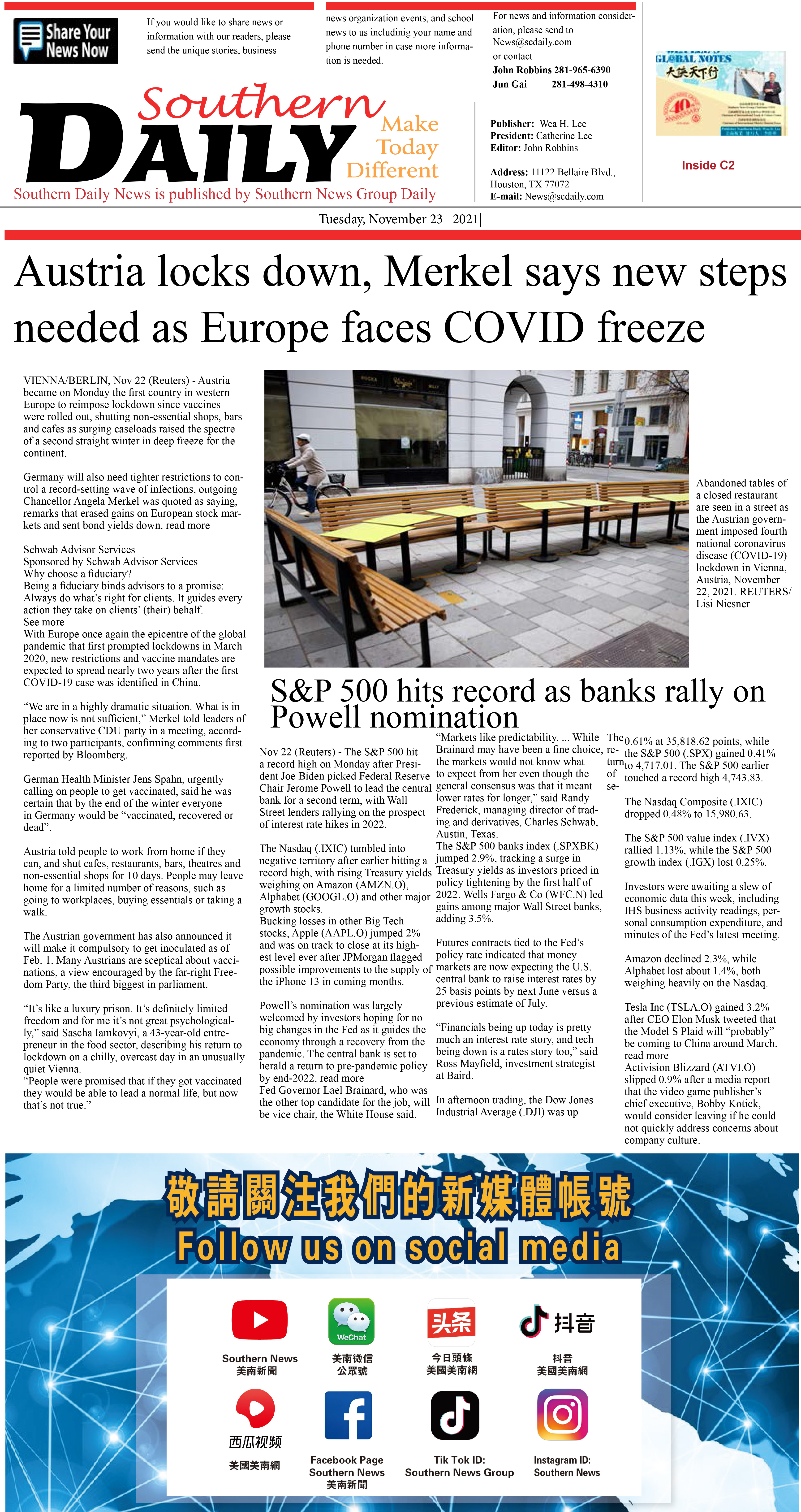211121 Epaper

 A Section B SectionC Section D Section